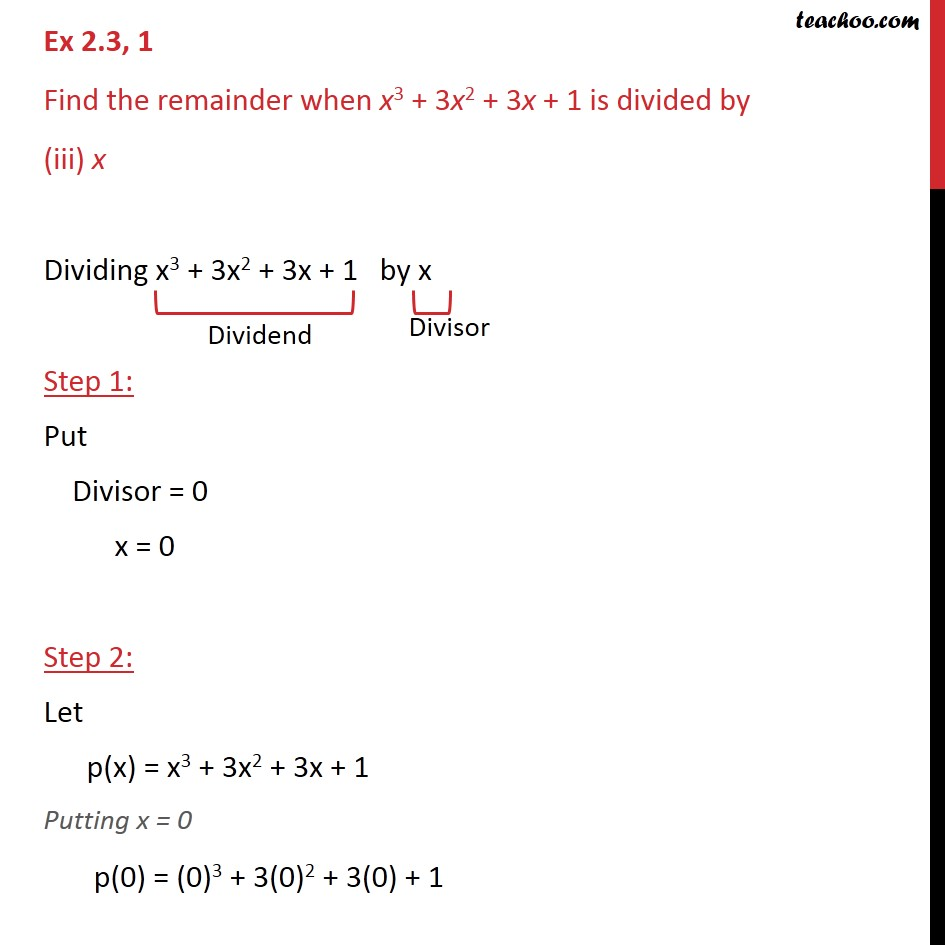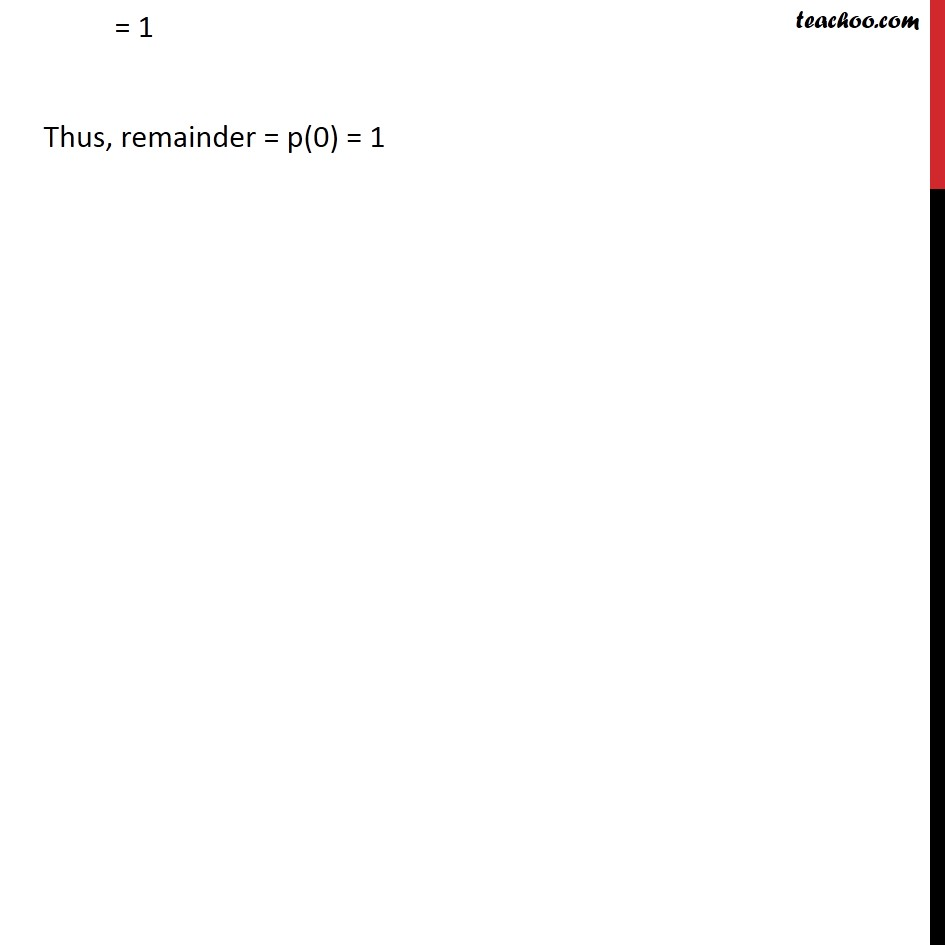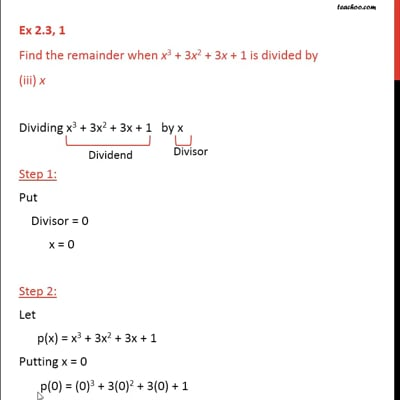Ex 2.3

Chapter 2 Class 9 Polynomials
Serial order wiseThis video is only available for Teachoo black users

Maths Crash Course - Live lectures + all videos + Real time Doubt solving!

### Transcript

Ex 2.3, 1 Find the remainder when x3 + 3x2 + 3x + 1 is divided by (iii) x Dividing x3 + 3x2 + 3x + 1 by x Step 1: Put Divisor = 0 x = 0 Step 2: Let p(x) = x3 + 3x2 + 3x + 1 Putting x = 0 p(0) = (0)3 + 3(0)2 + 3(0) + 1 = 1 Thus, remainder = p(0) = 1